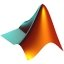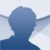# MATLAB Mac

R2019a

## MATLAB is one of the most powerful mathematical calculation tools available. Download MATLAB and get hold of this software to work with maths calculations

MATLAB is the most complete, stable and reliable program in the world for the development of mathematical processes. It has hundreds of different utilities which can be used to carry out any study in the mathematical field, from solving simple functions, to the most complex engineering calculations.

### Main features of MATLAB

• Tool for the creation of 2D and 3D graphics.
• Modular workflow system (functionalities can be expanded by means of plug-ins).
• Project management.
• Integrated development environment specially developed for matrix calculus.

Together with the development it is possible to use various programming languages like Pascal, C++, Visual Basic or Java, in such a way that it's possible to automate every kind of process and thus make it easier to work with multiple factors.

Download MATLAB and discover the most powerful weapon in the world of maths.